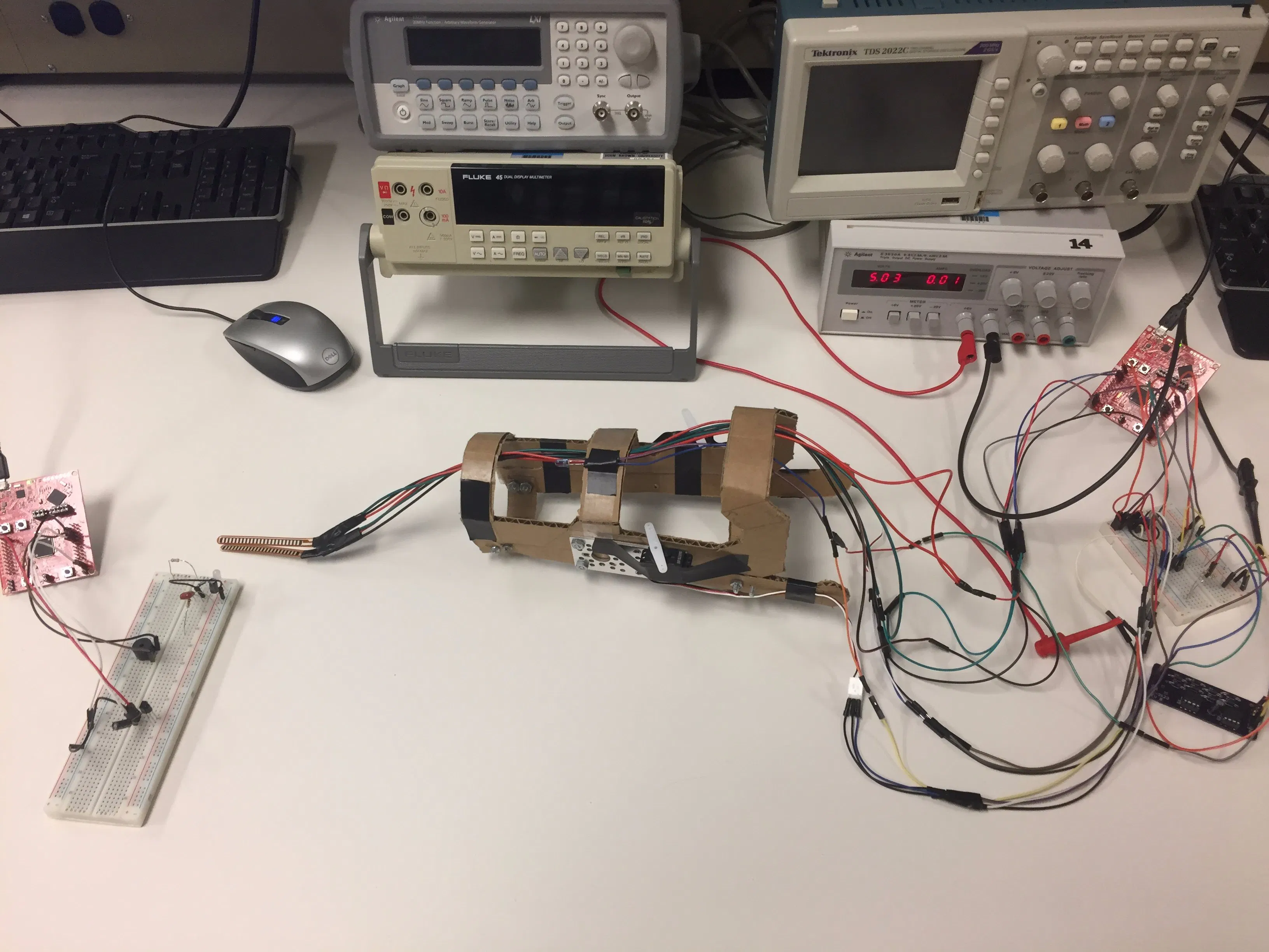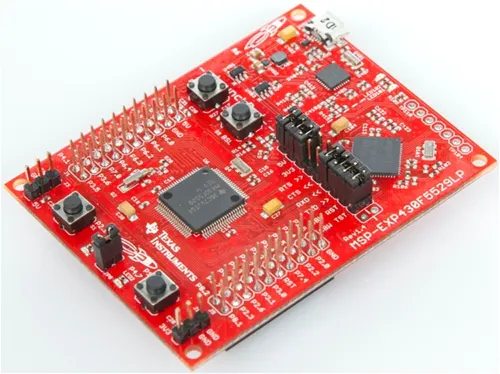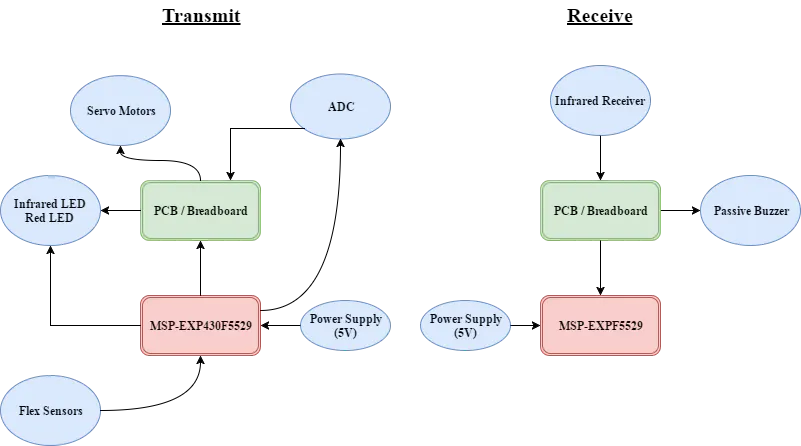# Mounted Infrared LED Laser Tag System

A laser tag system that uses flex sensors to control an infrared LED transmitter.

IntermediateShowcase (no instructions)5 hours626## Things used in this project

### Hardware components×2

### Hand tools and fabrication machinesSoldering iron (generic)

## Schematics

### Block Diagram

System Block diagram## Code

### Transmit Code for Infrared LED

C/C++
Code used to convert flex sensor analog voltage into data to control the infrared LED.
```//This program is the transmission portion of Jackson Love's final project.
//The program will read in a analog voltage from two flex sensors
//and control an infrared LED and two servo motors based on the threshold values.
//This program uses a motor driver to step up the current to operate the infrared LED.
#include <msp430.h>
#define Motor BIT2 //P1.2 is designated for the two motors
#define RedLED BIT4 // P1.4 designated for the test LED
#define InfLED BIT3 // P1.3 for the infrared LED
#define Button BIT1
#define Flex1  BIT0
#define Flex2  BIT1
const float VCC = 5.08; // 5V pin
const float R1_Divisor = 46120; //Flex sensor 1 divisor
const float R2_Divisor = 46520; //Flex sensor 2 divisor
const float R1StraightResistance = 28190; //Flex sensor 1 straight resistance
const float R2StraightResistance = 30770; //Flex sensor 2 straight resistance
const float R1BendResistance = 120000; //Max resistance value for R1
const float R2BendResistance = 125000; //Max resistance value for R2
int Angle = 1500;	//initial position.
int degree90 = 1600; //90 degrees. Neutral position
int degree45 = 2200;//45 degrees. Position 1
int motorOn = 1;//variable that tracks whether motor is on or off
int state = 0; //state machine variable that tells if at position 0 or 1
//position 0: neutral
//position 1: rotates right

void main(void)
{
WDTCTL = WDTPW+WDTHOLD;                   // Stop watchdog timer
P6SEL = Flex1 + Flex2;                             // Enable A/D channel inputs
WDTCTL = WDTPW + WDTHOLD;    // Stop watchdog timer
P1DIR |= BIT2 + RedLED+ InfLED; //Motors, Red LED, and Infrared set as outputs
P2REN |= Button;
P2OUT |= Button;
P1REN |= Button;            // enable pull-up resistor for switch
P1OUT |= Button;            // enable pull-up resistor for S2 (P1.3)
P1IE = Button;              // enable P1.3 interrupt
P1IES= Button;
P1IFG = 0x00;                // clears interrupt flag
TA0CCTL0 = CCIE;             // CCR0 interrupt enabled
TA0CCR0  = 5000;            // Set count threshold
TA0CTL    = TASSEL_1 + MC_0;  // SMCLK, up mode (starts timer!)
TA1CCTL0 = CCIE;             // CCR0 interrupt enabled
TA1CCR0  = 1500;            // Set count threshold
TA1CTL    = TASSEL_2 + MC_1;  // SMCLK, up mode (starts timer!)
__enable_interrupt();        // enables selected interupts

while(1) // infinitely loop
{

if(ADCresults < 2500) // if flex sensor 1 analog reading is less than 2500
{
P1OUT ^= RedLED; //turn on the Red LED as long as sensor is bent
P1OUT ^= InfLED; //turn on the infrared LED as long as sensor is bent
}
else
{
P1OUT &= ~InfLED; //turns off the red LED
P1OUT	&= ~RedLED; //turns off the infrared LED
}

if (ADCresults < 2500) // if flex sensor 2 analog reading is less than 2500
{
Angle = degree45;//move motor to angle of 90
state = 1;//change state
}
else
{
Angle = degree90;//rotate motor 45 degrees

}

}
}

#pragma vector=TIMER1_A0_VECTOR
__interrupt void Timer_A1 (void) // Timer A0 interrupt service routine
{   // the TACCR0 CCIFG flag is automatically reset
if (motorOn == 1)//statement for motor on
{
P1OUT |= BIT2;//sets P1.2 as output, turns motor on
TA1CCR0  = Angle;   // Sets max counter to Angle
motorOn = 0;//set to 0 for motor off state
}
else
{
motorOn = 1;
P1OUT &= ~BIT2; //turns motor off
TA1CCR0  = 20000;//delay for 20 millisecond
}
}
//reads the analog values and assigns them to the results buffer
#if defined(__TI_COMPILER_VERSION__) || defined(__IAR_SYSTEMS_ICC__)
#elif defined(__GNUC__)
#else
#error Compiler not supported!
#endif
{
{
case  0: break;                           // Vector  0:  No interrupt
case  2: break;                           // Vector  2:  ADC overflow
case  4: break;                           // Vector  4:  ADC timing overflow
case  6: break;                           // Vector  6:  ADC12IFG0
case  8: break;                           // Vector  8:  ADC12IFG1
case 10: break;                           // Vector 10:  ADC12IFG2
case 12:                                  // Vector 12:  ADC12IFG3
case 14: break;                           // Vector 14:  ADC12IFG4
case 16: break;                           // Vector 16:  ADC12IFG5
case 18: break;                           // Vector 18:  ADC12IFG6
case 20: break;                           // Vector 20:  ADC12IFG7
case 22: break;                           // Vector 22:  ADC12IFG8
case 24: break;                           // Vector 24:  ADC12IFG9
case 26: break;                           // Vector 26:  ADC12IFG10
case 28: break;                           // Vector 28:  ADC12IFG11
case 30: break;                           // Vector 30:  ADC12IFG12
case 32: break;                           // Vector 32:  ADC12IFG13
case 34: break;                           // Vector 34:  ADC12IFG14
default: break;
}
}
```

### Receiving Circuit Code

C/C++
Code used with an Infrared Receiver and passive buzzer.
```#include  <msp430.h>
#define RedLED BIT0
#define GreenLED BIT6
#define Button BIT3 // output of infrared receiver
#define SPEAKERLOW BIT4 //negative piezzo
#define SPEAKERHIGH BIT5 //positive piezzo
int enableBuzzer = 0;
void playNote(unsigned char pitch);
void playNoteForDuration(unsigned char pitch, unsigned char duration);

void main(void) {
WDTCTL = WDTPW + WDTHOLD;       // Stop watchdog timer
P1DIR |= SPEAKERLOW | SPEAKERHIGH;		//sets the two LEDs to output
P1REN |= Button;
P1IE = Button;	//enable interrupt for Button
P1IES = Button;	//initializes the interrupt on the clock edge select
P1OUT |= Button;			//sets Button as an output
TA0CCR0 = 3000;              // Upper limit of 3000 cycles
TA0CCTL0 = CCIE;             // enable the interrupts from TimerA0 CC0
_enable_interrupts();
//note that TACTL is not initialized. It will be called in the PORT1 interrupt

while (1)
{
if (enableBuzzer==1)
{

playNoteForDuration('c',1);
enableBuzzer=0;
P1IE = Button;
}
}
}
#pragma vector = TIMER0_A0_VECTOR	//timer interrupt
__interrupt void TA0_ISR(void)
{

enableBuzzer = 1;	//toggles the Green LED

TA0CTL = MC_0;//then sets the control to MC_0 to stop counting
}
//if the Button is pressed, PORT1 interrupt starts
//normally, TACTL is MC_0, so this interrupt sets TACTL to MC_1
//if the button is pressed, Timer A will begin counting up to 3000
//This way the timer is only started when the button is pressed
#pragma vector = PORT1_VECTOR
__interrupt void PORT1_ISR(void)
{
if (!(TA0CTL & MC_1)){	//if Timer A control is not set to MC_1
TA0CTL = TASSEL_2|MC_1|ID_2|TACLR;	//sets TACTL is set to MC_1
P1IFG = 0;	//clears flag
}
P1IE &= ~Button;
P1IFG = 0;	//clears flag
}

void playNote(unsigned char pitch) //Function to play the array of notes
{
P1OUT &= ~SPEAKERLOW;   //grounds the speaker
P1OUT |= SPEAKERHIGH; //Speaker will constantly be changing therefore creating a sound
int P;  //local variable for Pitch
int L = 15000;  //sets the length of the note to be played
int j;
int i;
int h;
switch (pitch) // note that the frequency to loop ratio is 28923.8/Hz = loop number
{//a range of 7 notes are used in this array
case ('c'):  //middle c note in the 4th octave
P = 360;  //calculated frequency for c
L = L / P; //This gives each note a common time. Syncs with the chip clock
break;
case ('d'):  //d note in the 4th octave
P = 232;
L = L / P;
break;
case ('e'):  //e note in the 4th octave
P = 206;
L = L / P;
break;
case ('f'):  //f note in the 4th octave
P = 195;
L = L / P;
break;
case ('g'):  //g note in the 4th octave
P = 174;
L = L / P;
break;
case ('a'):  //a note in the 4th octave
P = 155;
L = L / P;
break;
case ('b'):  //b note below middle c (3rd octave)
P = 276;
L = L / P;
break;
case ('C'):  //c note in the 5th octave
P = 130;
L = L / P;
break;
case ('r'):  //rest case. This allows a musical break in between notes
P = 0;
L = L / 4;  //specifically catered to be a different loop cycle
break;
default:
P = 0;
}
for (h = 5; h > 0; h--) { //multiplies the Length (L) to a play at a longer duration
for (j = L; j > 0; j--)  //sets j = L (15000) to loop 15000 times
{
if (P != 0) //when P =0, there is not a set frequency, but is inaudible to hear
{ //by setting P not to 0, this will read the rest case as a pause, not a frequency
P1OUT ^= SPEAKERLOW;    //speakers will play a frequency.
P1OUT ^= SPEAKERHIGH;
}
for (i = P; i > 0; i--)
; //P set to an integer to keep the frequency everytime. Otherwise P will be 0 constantly as it cycles
}
}
}

void playNoteForDuration(unsigned char pitch, unsigned char duration) //Duration function for playing the note
{ //this function will multiply the length of the note in playNote function for a duration multiplier set in the array
int i;    //sets i = duration intitially
for (i = duration; i > 0; i--)
playNote(pitch); //passes in pitch as it cycles through the array
}
```

## Credits

### Jackson Love

1 project • 0 followers